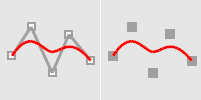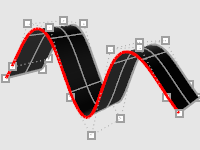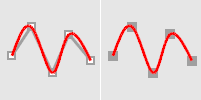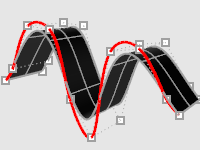# CurveThroughPolylineHistory enabled.

The CurveThroughPolyline command creates a curve from vertex points of a polyline.

Steps

Select polylines.

Command-line options

Degree

Specifies the degree of the curve (or surface).

When drawing a high-degree curve, the output curve will not be the degree you request unless there is at least one more control point than the degree.

CurveType

ControlPoint

The curve's control points pass through the vertices, points, or control points.Curve control points match input.Resulting extruded surface.

Interpolated

The curve passes through the vertices, points, or control points.Curve matches input.Resulting extruded surface.

Knots

Determines how an interpolated curve is parameterized. When the spacing between the picked points is equal for the whole curve, all three parameterizations generate the same curve.

When you draw an interpolated curve, the points you pick are converted into knot values on the curve. The parameterization means how the intervals between knots are chosen.

Uniform

The knot spacing is always 1 and is not based on the physical spacing of the points. Uniform parameterization can be used if the point spacing is roughly equal and it is desirable to draw several curves that have the same parameterization. This is possible only with uniform curves (unless you rebuild the curves). Uniform guarantees that every control point affects the surface exactly the same way, no matter how much the surface is edited.

Chord

The spacing between the picked points is used for the knot spacing. This makes curves that have widely varying point spacing behave better than uniform curves.

SqrtChrd

The square root of the spacing between picked points is used for the knot spacing.